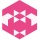Home
IT Knowledge
Inspiration
Languages
EN

# Java - how to use Iterator with ArrayList

0 points
Created by:Violet-Hoffman
232

In this article, we would like to show you how to use iterator with ArrayList in Java.

Quick solution:

``````Iterator<String> myIterator = myArrayList.iterator();

while (myIterator.hasNext()) {
System.out.print(myIterator.next() + " ");
}``````

## Introduction

`Iterator` is an object that can be used to loop through collections. In this article, we will focus on the ArrayList collection and loop through it.

### Create iterator

To use Iterator, the first thing we need to do is obtain it from a Collection. To do so, we use `iterator()` method in the following way:

``````List<String> myArrayList = ...
Iterator<String> myIterator = myArrayList.iterator();
``````

### Iterator methods

• `hasNext()` - checks if there is at least one element left to iterate over, it's commonly used as a condition in while loops.
• `next()` - steps over the next element and obtains it.

## Practical example

In this example, we create an iterator for `letters` ArrayList to display all the elements in the proper order.

``````import java.util.*;

public class Example {

public static void main(String[] args) {
List<String> letters = new ArrayList<>();

// create an iterator
Iterator<String> myIterator = letters.iterator();

// display values after iterating through the ArrayList
System.out.println("The iterator values of the list are: ");
while (myIterator.hasNext()) {
System.out.print(myIterator.next() + " ");
}
}
}``````

Output:

``````The iterator values of the list are:
A B C ``````